No version for distro dashing. Known supported distros are highlighted in the buttons above.
No version for distro crystal. Known supported distros are highlighted in the buttons above.
No version for distro bouncy. Known supported distros are highlighted in the buttons above.

Package Summary

 Tags No category tags. Version 0.2.5 License BSD Build type CATKIN Use RECOMMENDED

Repository Summary

 Checkout URI https://github.com/locusrobotics/robot_navigation.git VCS Type git VCS Version tf2 Last Updated 2019-06-07 Dev Status DEVELOPED Released RELEASED

Package Description

Implementation of dlux_global_planner plugin interfaces.

Maintainers

• David V. Lu!!

dlux_plugins

Be sure to start with the documentation for `dlux_global_planner`.

PotentialCalculators

The two key questions in calculating the potential are 1. What order should we calculate the potential in? 2. What value should we assign the the potential?

There are two potential calculators provided.

Dijkstra

`dlux_plugins::Dijkstra` calculates the potential in full breadth first order, starting at the goal. The values that it calculates are from the kernel function. Since the kernel function uses the minimum potential of two of the cell's four neighbors, and breadth-first search guarantees the potential will not ever decrease, each potential is stable, i.e. once a value is calculated, that value will not change, i.e. it will not get a new neighbor with a lower potential.

AStar

`dlux_plugins::AStar` uses a heuristic to guide the order in which it calculates the potential. The heuristic can be either the Manhattan distance or the Cartesian distance (depending on the `manhattan_heuristic` parameter; default is false). You can also choose whether the calculated values use the kernel function, or are straightforward "one-neighbor" potentials (using the `use_kernel` parameter, default is true).

If using the kernel function, however, the values can be unstable. Because the calculated potentials are not non-decreasing (i.e. if it reaches a dead end, it will go back and compute lower potentials again), the algorithm will sometimes need to decrease the value of previously calculated potential. As a result, the cell will be requeued, and all cells that depended on the previous value will also need to be recalculated. Thus, `AStar` may end up expanding more cells than `Dijkstra`! For an illustrated example of this, see this presentation

One way to prevent this is to only change the potential if the value changes by a certain amount. This is implemented with the `minimum_requeue_change` (default is 1.0) such that if the potential changes by less than that threshold, the change in potential will be ignored, and the cell will not be requeued. While this results in slightly different potentials being calculated, the time savings can justify "good enough" potentials.

Traceback Algorithms

There are three traceback algorithms provided. * `dlux_plugins::VonNeumannPath` - Moves from cell to cell using only the cell's four neighbors. * `dlux_plugins::GridPath` - Moves from cell to cell using the cell's eight neighbors. * `dlux_plugins::GradientPath` - Uses the potential to calculate a gradient not constrained by the grid.

At each cell it visits it calculates a two-dimensional gradient based on the potentials in the neighboring cells. It then uses that gradient to calculate the next point on the path `step_size` away (default 0.5). The result is often shorter, smoother paths. This was the default `navfn` behavior.

There are two additional parameters that step from the `navfn` roots of this code. First, `lethal_cost` (default=250.0) is a constant used in calculating gradients near lethal obstacles. There is also `grid_step_near_high` (default false). In the original `navfn` implementation, if the current cell in the traceback was adjacent to a uncalculated potential, it would revert back to moving directly to one of the eight neighbors (a.k.a. a grid step). However, this is not strictly necessary, and is turned off by default.

One final parameter is `iteration_factor` (default 4.0). As a safeguard against infinitely iterating through local minima in the potentials, we limit the number of steps we take, relative to the size of the costmap. The maximum number of iterations (and therefore steps in the path) is `width * height * iteration_factor`.

Example Paths

Different combinations of plugins and parameters will result in different paths.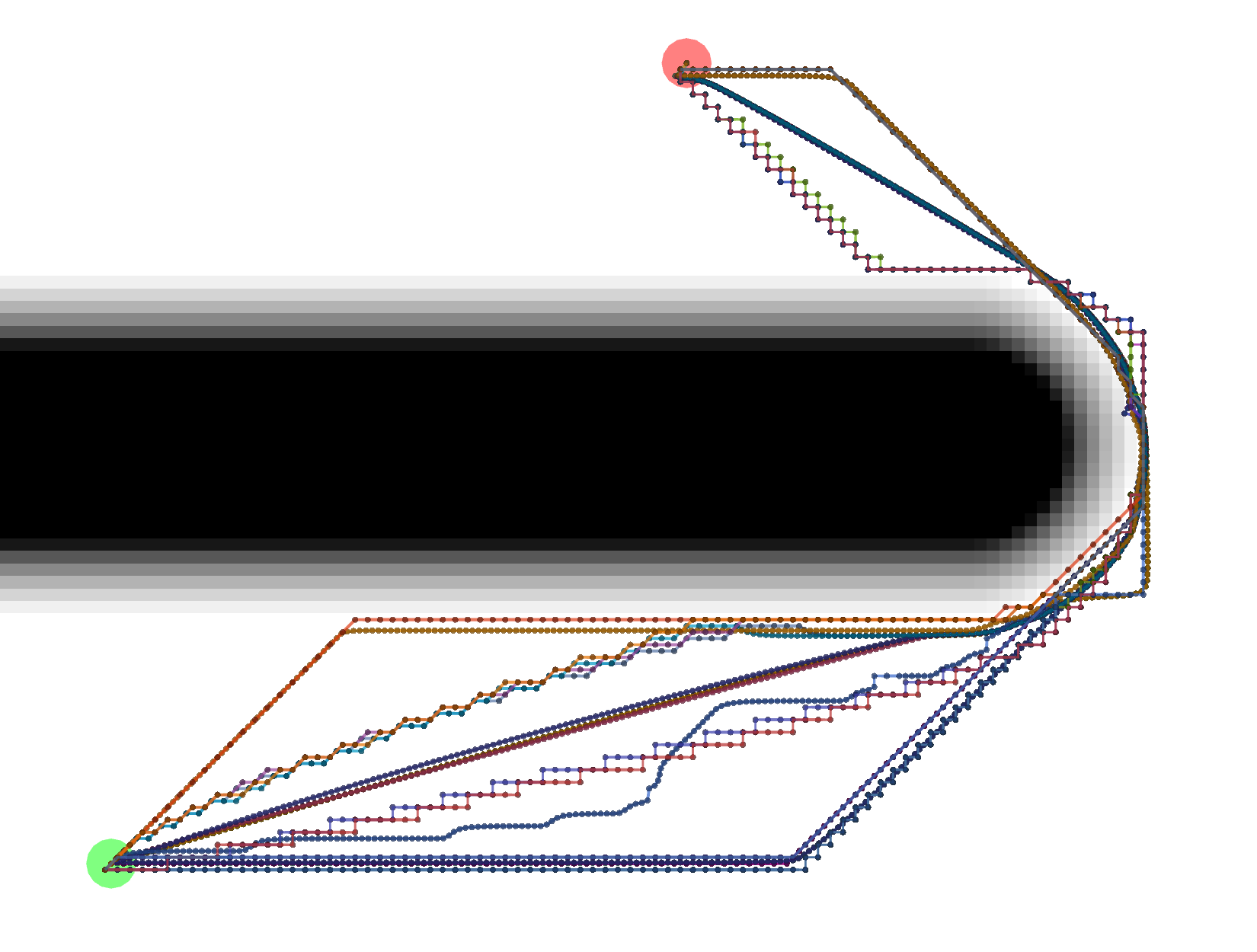Start location in green, goal in red.

Example Tracebacks

Let's start by looking at the different possible Tracebacks.

VonNeumann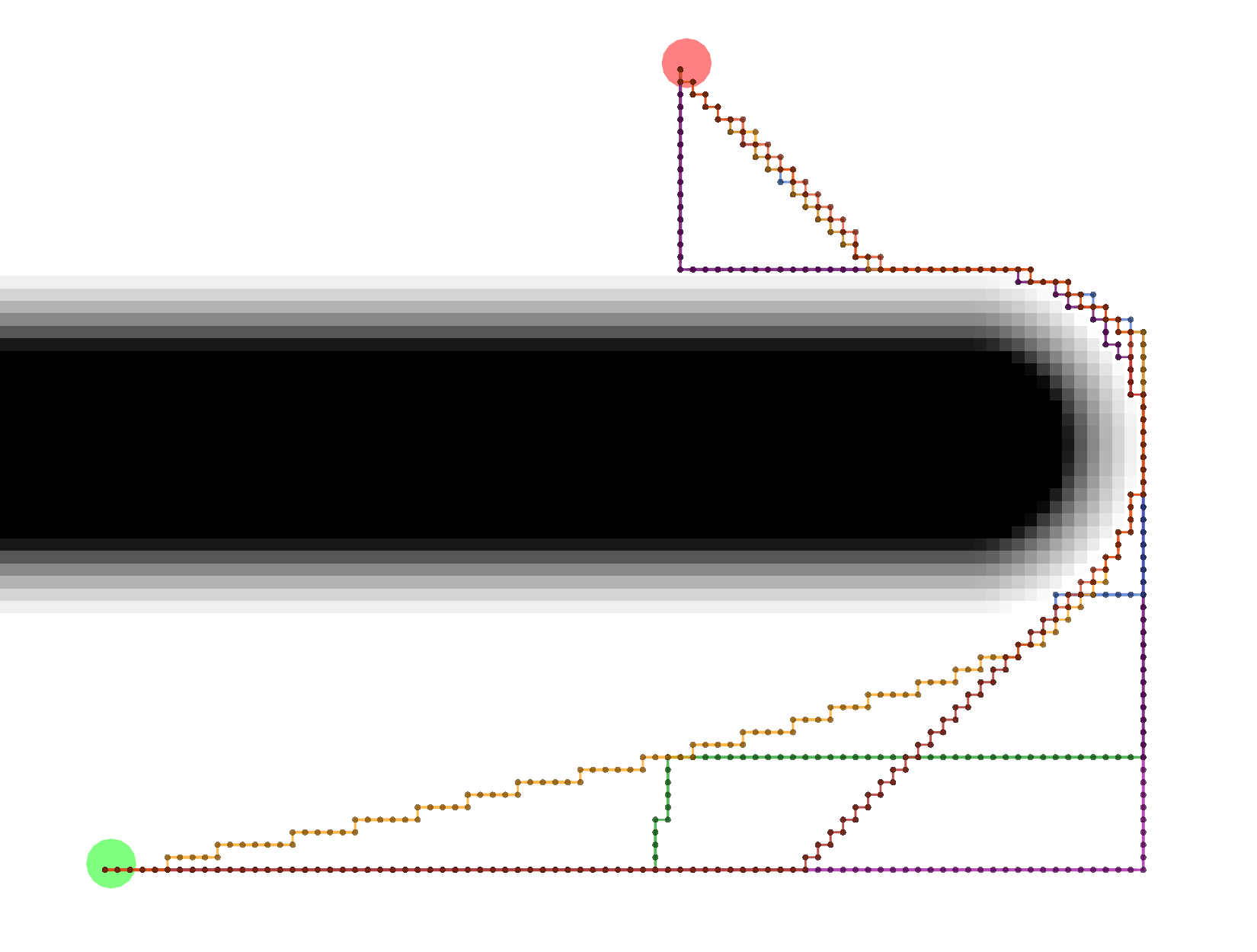All of these paths actually have the same exact length.

Grid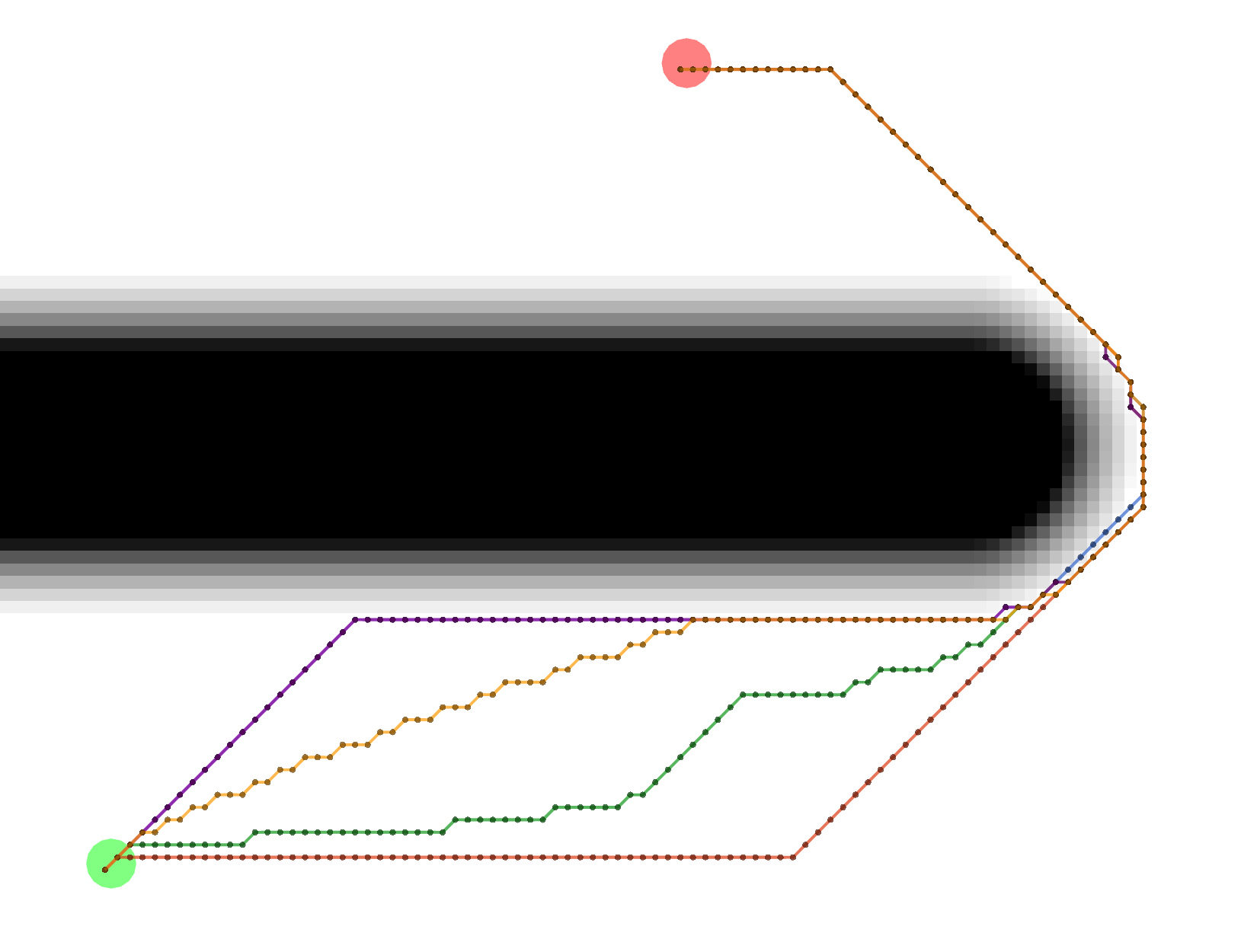All of these Grid paths are a bit shorter than the VonNeumann paths, but since they are constrained to certain diagonals, they are not optimally short.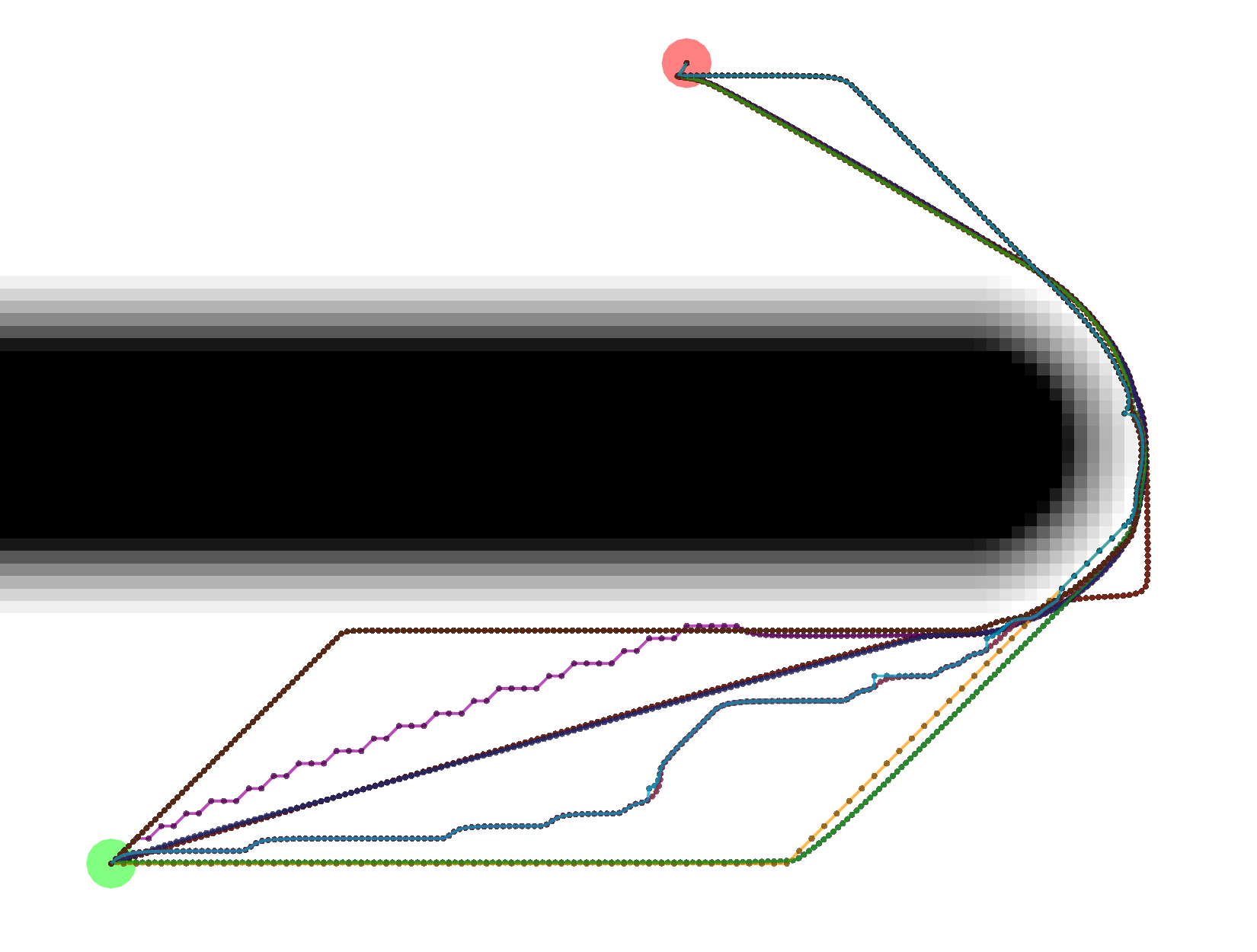Gradient paths tend to be the shortest, but are a bit more complicated to compute. Some of them look like Grid paths either because of the potentials that were calculated or because the Gradient path sometimes uses grid-constrained steps as a backup.

Example Potential Calculations

Each potential calculation is shown with the four tracebacks (there's two Gradient paths with different values of `grid_step_near_high`)

DijkstraAs expected, Dijkstra calculates the most potentials.

AStar with Manhattan Heuristic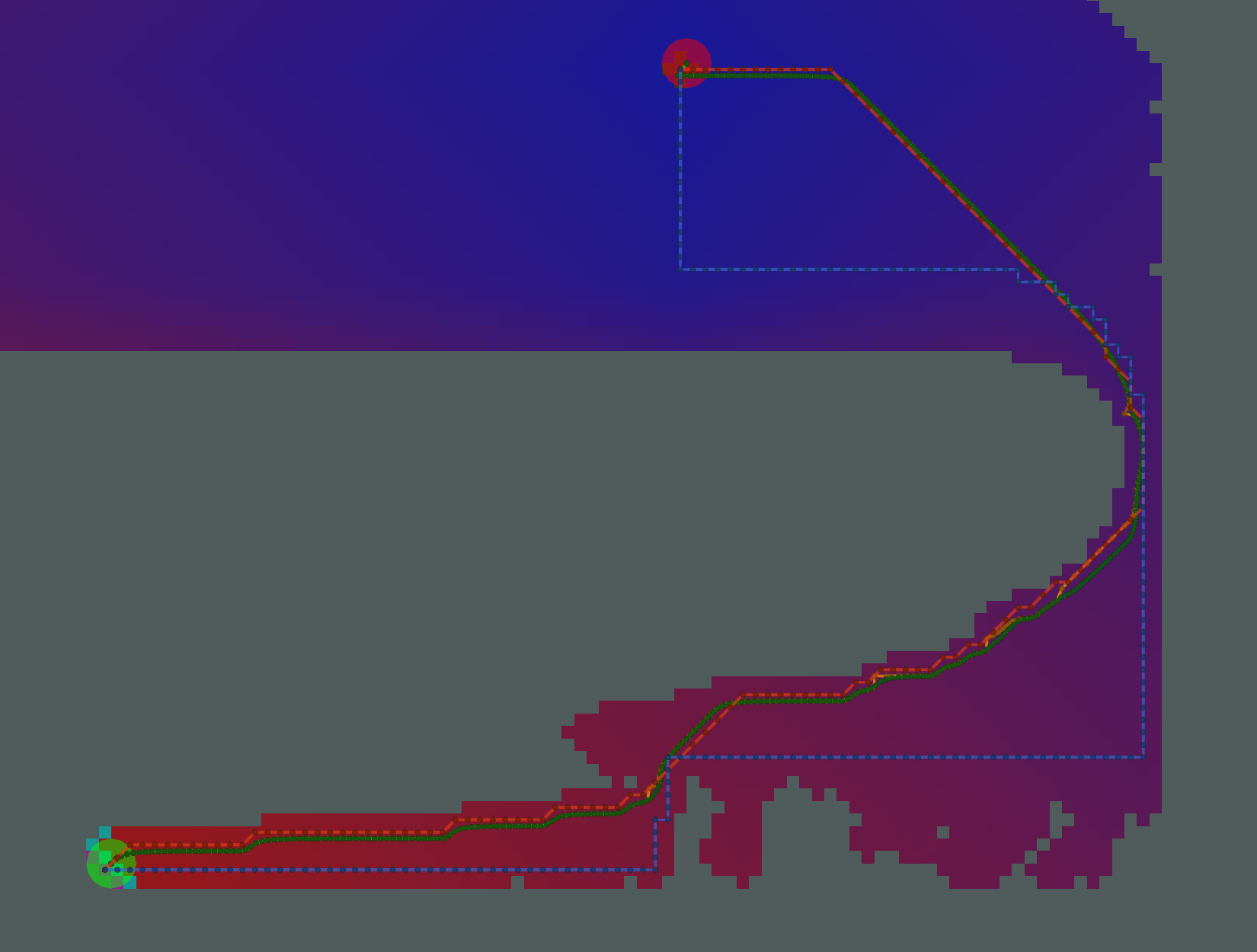AStar calculates fewer potentials here. However, since there are many cells on the bottom of the image with the same heuristic (i.e. same Manhattan distance to the start and same Manhattan distance to the goal), the resulting pattern of calculated and uncalculated potentials looks random.

AStar with Euclidean Heuristic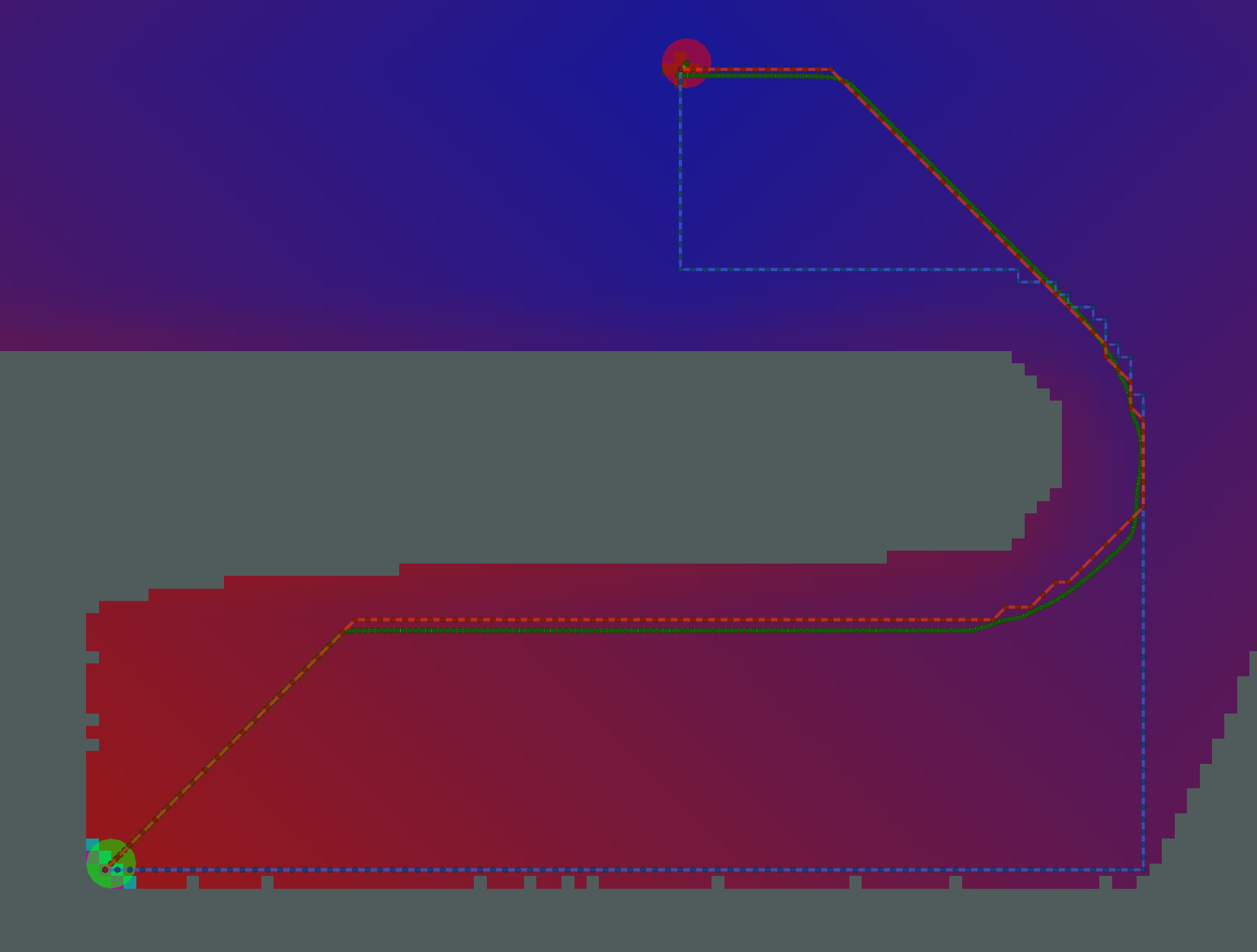The pattern of calculated/uncalculated potentials here is less random. However, the Grid and Gradient paths are nearly the same because the kernel function is not used.

AStar with Kernel and Manhattan Heuristic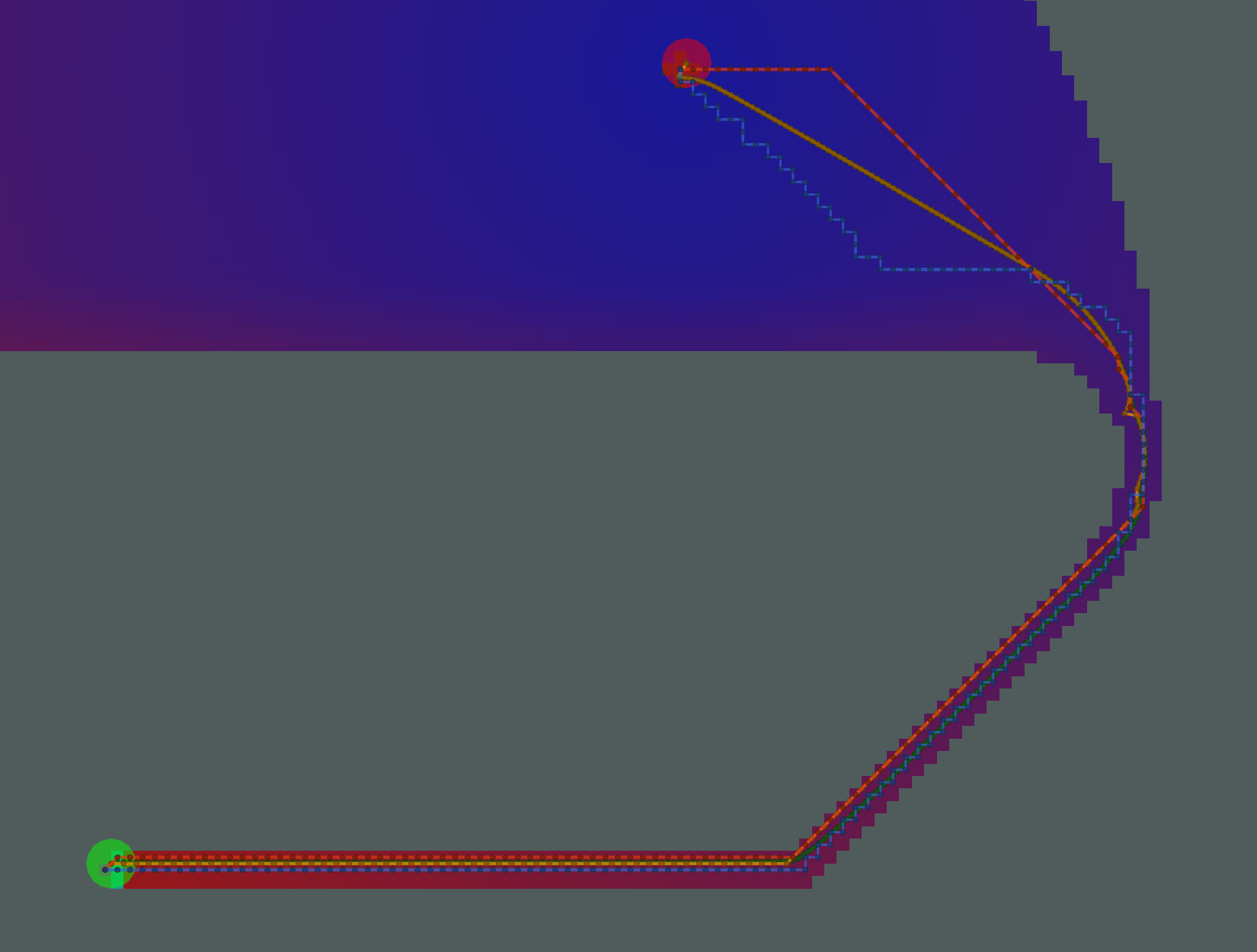Many fewer potentials expanded here, but the path is not sufficiently short, due to the limited potentials to traceback through.

AStar with Kernel and Euclidean Heuristic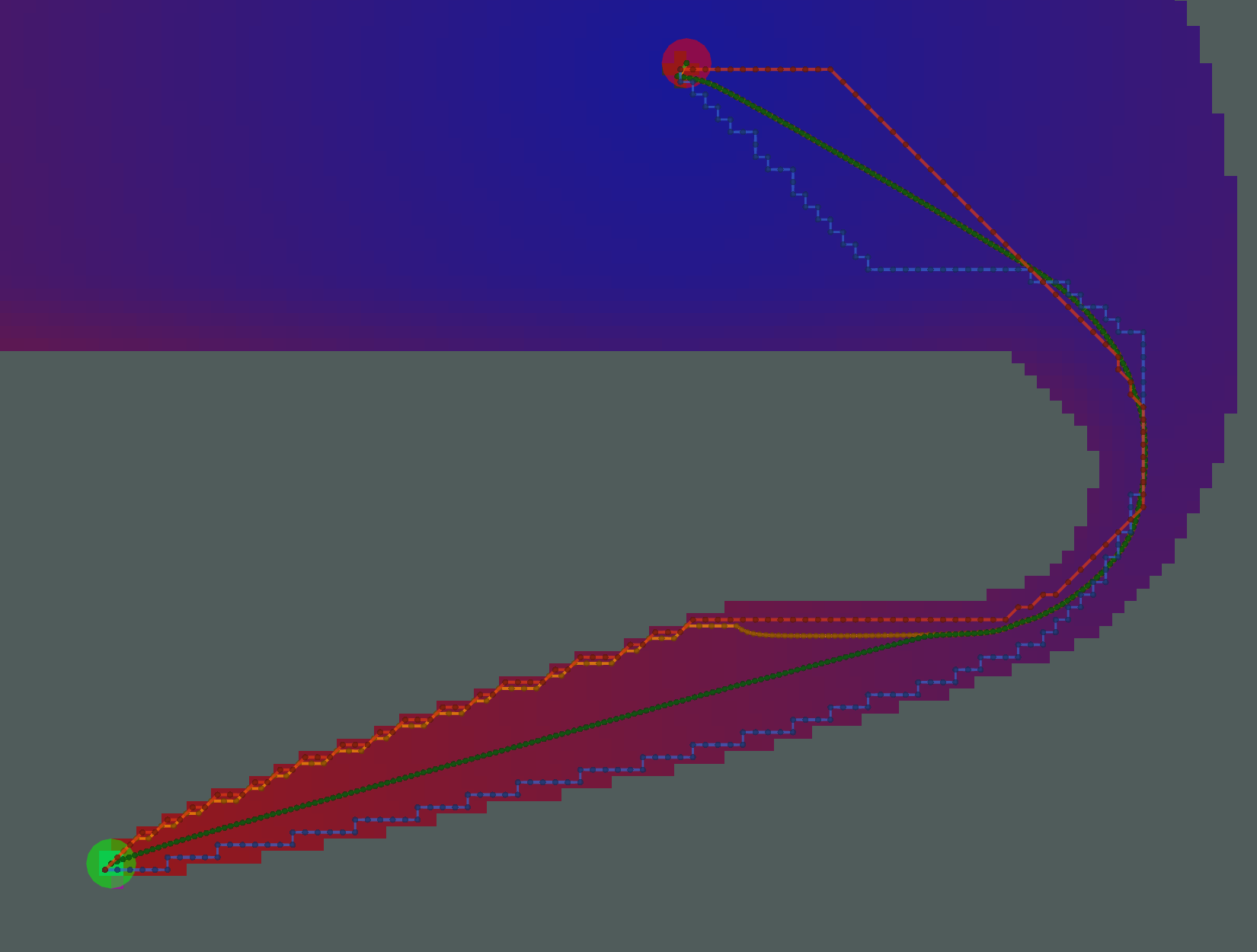Here, we have an example where the two Gradient paths (orange and green) are noticeably different. The orange path has `grid_step_near_high=true`, and gets "stuck" along the border of the potential. The green path has `grid_step_near_high=false` and results in a much smoother path.

Analysis

There is a tradeoff between number of cells expanded / potentials calcualted and the resulting length of the path. Ideally, you would minimize each. For the particular start/goal shown in the examples above, we can plot the number of cells and length of the path.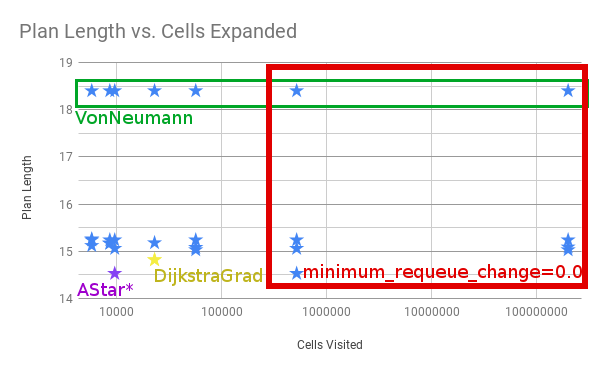Four things to call out. * All of the examples in the red box with the most cells visited have `minimum_requeue_change=0.0` * All the longest paths in the green box use the `VonNeumann` traceback. * `NavFn` and `Dijkstra + GradientPath` result in the yellow star. * The absolute shortest path is marked in purple as `AStar*`, meaning AStar using the Kernel and Euclidean Heuristic. It's shorter than Dijkstra in this one particular example because the `DijkstraGrad` path takes one extra little loop, as shown below.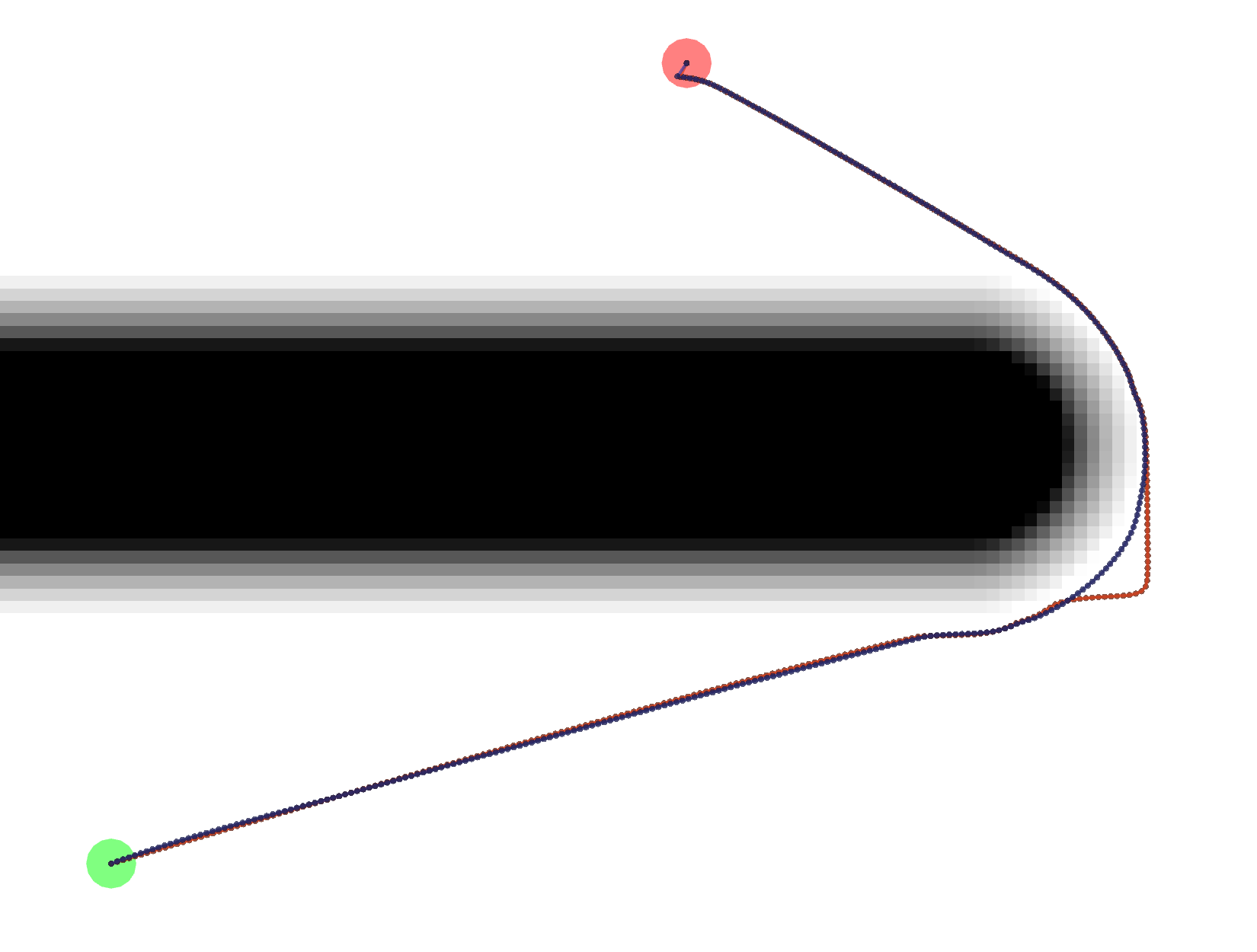The exact reason for the difference in paths is unknown at this time.

Tests

`test/planner_test.cpp` makes use of the `global_planner_tests` package to run a series of tests on some key combinations of plugins and parameters. `test/full_planner_test.cpp` is not run by default and runs ALL combinations.

Demo

You can also run `roslaunch dlux_plugins node_test.launch` to run multiple instances of the `planner_node.cpp` which will show the variation between the different paths as shown above.

CHANGELOG
No CHANGELOG found.

Wiki Tutorials

See ROS Wiki Tutorials for more details.

Source Tutorials

Not currently indexed.

System Dependencies

No direct system dependencies.

Messages

No message files found.

Services

No service files found

Recent questions tagged dlux_plugins at answers.ros.org

Package Summary

 Tags No category tags. Version 0.2.5 License BSD Build type CATKIN Use RECOMMENDED

Repository Summary

 Checkout URI https://github.com/locusrobotics/robot_navigation.git VCS Type git VCS Version master Last Updated 2019-06-24 Dev Status DEVELOPED Released RELEASED

Package Description

Implementation of dlux_global_planner plugin interfaces.

Maintainers

• David V. Lu!!

dlux_plugins

Be sure to start with the documentation for `dlux_global_planner`.

PotentialCalculators

The two key questions in calculating the potential are 1. What order should we calculate the potential in? 2. What value should we assign the the potential?

There are two potential calculators provided.

Dijkstra

`dlux_plugins::Dijkstra` calculates the potential in full breadth first order, starting at the goal. The values that it calculates are from the kernel function. Since the kernel function uses the minimum potential of two of the cell's four neighbors, and breadth-first search guarantees the potential will not ever decrease, each potential is stable, i.e. once a value is calculated, that value will not change, i.e. it will not get a new neighbor with a lower potential.

AStar

`dlux_plugins::AStar` uses a heuristic to guide the order in which it calculates the potential. The heuristic can be either the Manhattan distance or the Cartesian distance (depending on the `manhattan_heuristic` parameter; default is false). You can also choose whether the calculated values use the kernel function, or are straightforward "one-neighbor" potentials (using the `use_kernel` parameter, default is true).

If using the kernel function, however, the values can be unstable. Because the calculated potentials are not non-decreasing (i.e. if it reaches a dead end, it will go back and compute lower potentials again), the algorithm will sometimes need to decrease the value of previously calculated potential. As a result, the cell will be requeued, and all cells that depended on the previous value will also need to be recalculated. Thus, `AStar` may end up expanding more cells than `Dijkstra`! For an illustrated example of this, see this presentation

One way to prevent this is to only change the potential if the value changes by a certain amount. This is implemented with the `minimum_requeue_change` (default is 1.0) such that if the potential changes by less than that threshold, the change in potential will be ignored, and the cell will not be requeued. While this results in slightly different potentials being calculated, the time savings can justify "good enough" potentials.

Traceback Algorithms

There are three traceback algorithms provided. * `dlux_plugins::VonNeumannPath` - Moves from cell to cell using only the cell's four neighbors. * `dlux_plugins::GridPath` - Moves from cell to cell using the cell's eight neighbors. * `dlux_plugins::GradientPath` - Uses the potential to calculate a gradient not constrained by the grid.

At each cell it visits it calculates a two-dimensional gradient based on the potentials in the neighboring cells. It then uses that gradient to calculate the next point on the path `step_size` away (default 0.5). The result is often shorter, smoother paths. This was the default `navfn` behavior.

There are two additional parameters that step from the `navfn` roots of this code. First, `lethal_cost` (default=250.0) is a constant used in calculating gradients near lethal obstacles. There is also `grid_step_near_high` (default false). In the original `navfn` implementation, if the current cell in the traceback was adjacent to a uncalculated potential, it would revert back to moving directly to one of the eight neighbors (a.k.a. a grid step). However, this is not strictly necessary, and is turned off by default.

One final parameter is `iteration_factor` (default 4.0). As a safeguard against infinitely iterating through local minima in the potentials, we limit the number of steps we take, relative to the size of the costmap. The maximum number of iterations (and therefore steps in the path) is `width * height * iteration_factor`.

Example Paths

Different combinations of plugins and parameters will result in different paths.Start location in green, goal in red.

Example Tracebacks

Let's start by looking at the different possible Tracebacks.

VonNeumannAll of these paths actually have the same exact length.

GridAll of these Grid paths are a bit shorter than the VonNeumann paths, but since they are constrained to certain diagonals, they are not optimally short.Gradient paths tend to be the shortest, but are a bit more complicated to compute. Some of them look like Grid paths either because of the potentials that were calculated or because the Gradient path sometimes uses grid-constrained steps as a backup.

Example Potential Calculations

Each potential calculation is shown with the four tracebacks (there's two Gradient paths with different values of `grid_step_near_high`)

Dijkstra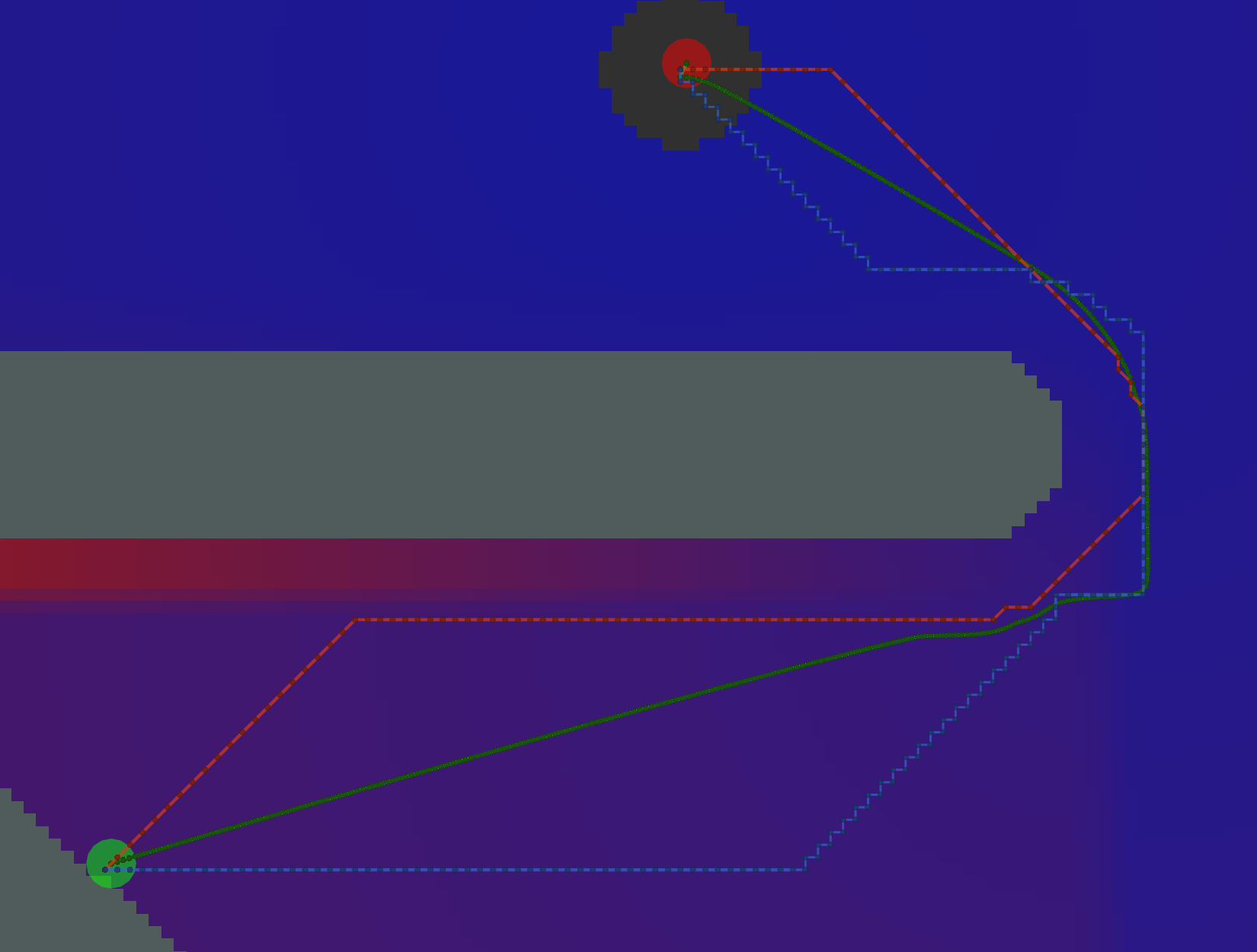As expected, Dijkstra calculates the most potentials.

AStar with Manhattan HeuristicAStar calculates fewer potentials here. However, since there are many cells on the bottom of the image with the same heuristic (i.e. same Manhattan distance to the start and same Manhattan distance to the goal), the resulting pattern of calculated and uncalculated potentials looks random.

AStar with Euclidean HeuristicThe pattern of calculated/uncalculated potentials here is less random. However, the Grid and Gradient paths are nearly the same because the kernel function is not used.

AStar with Kernel and Manhattan HeuristicMany fewer potentials expanded here, but the path is not sufficiently short, due to the limited potentials to traceback through.

AStar with Kernel and Euclidean HeuristicHere, we have an example where the two Gradient paths (orange and green) are noticeably different. The orange path has `grid_step_near_high=true`, and gets "stuck" along the border of the potential. The green path has `grid_step_near_high=false` and results in a much smoother path.

Analysis

There is a tradeoff between number of cells expanded / potentials calcualted and the resulting length of the path. Ideally, you would minimize each. For the particular start/goal shown in the examples above, we can plot the number of cells and length of the path.Four things to call out. * All of the examples in the red box with the most cells visited have `minimum_requeue_change=0.0` * All the longest paths in the green box use the `VonNeumann` traceback. * `NavFn` and `Dijkstra + GradientPath` result in the yellow star. * The absolute shortest path is marked in purple as `AStar*`, meaning AStar using the Kernel and Euclidean Heuristic. It's shorter than Dijkstra in this one particular example because the `DijkstraGrad` path takes one extra little loop, as shown below.The exact reason for the difference in paths is unknown at this time.

Tests

`test/planner_test.cpp` makes use of the `global_planner_tests` package to run a series of tests on some key combinations of plugins and parameters. `test/full_planner_test.cpp` is not run by default and runs ALL combinations.

Demo

You can also run `roslaunch dlux_plugins node_test.launch` to run multiple instances of the `planner_node.cpp` which will show the variation between the different paths as shown above.

CHANGELOG
No CHANGELOG found.

Wiki Tutorials

See ROS Wiki Tutorials for more details.

Source Tutorials

Not currently indexed.

System Dependencies

No direct system dependencies.

Messages

No message files found.

Services

No service files found

Recent questions tagged dlux_plugins at answers.ros.org

Package Summary

 Tags No category tags. Version 0.2.5 License BSD Build type CATKIN Use RECOMMENDED

Repository Summary

 Checkout URI https://github.com/locusrobotics/robot_navigation.git VCS Type git VCS Version master Last Updated 2019-06-24 Dev Status DEVELOPED Released RELEASED

Package Description

Implementation of dlux_global_planner plugin interfaces.

Maintainers

• David V. Lu!!

dlux_plugins

Be sure to start with the documentation for `dlux_global_planner`.

PotentialCalculators

The two key questions in calculating the potential are 1. What order should we calculate the potential in? 2. What value should we assign the the potential?

There are two potential calculators provided.

Dijkstra

`dlux_plugins::Dijkstra` calculates the potential in full breadth first order, starting at the goal. The values that it calculates are from the kernel function. Since the kernel function uses the minimum potential of two of the cell's four neighbors, and breadth-first search guarantees the potential will not ever decrease, each potential is stable, i.e. once a value is calculated, that value will not change, i.e. it will not get a new neighbor with a lower potential.

AStar

`dlux_plugins::AStar` uses a heuristic to guide the order in which it calculates the potential. The heuristic can be either the Manhattan distance or the Cartesian distance (depending on the `manhattan_heuristic` parameter; default is false). You can also choose whether the calculated values use the kernel function, or are straightforward "one-neighbor" potentials (using the `use_kernel` parameter, default is true).

If using the kernel function, however, the values can be unstable. Because the calculated potentials are not non-decreasing (i.e. if it reaches a dead end, it will go back and compute lower potentials again), the algorithm will sometimes need to decrease the value of previously calculated potential. As a result, the cell will be requeued, and all cells that depended on the previous value will also need to be recalculated. Thus, `AStar` may end up expanding more cells than `Dijkstra`! For an illustrated example of this, see this presentation

One way to prevent this is to only change the potential if the value changes by a certain amount. This is implemented with the `minimum_requeue_change` (default is 1.0) such that if the potential changes by less than that threshold, the change in potential will be ignored, and the cell will not be requeued. While this results in slightly different potentials being calculated, the time savings can justify "good enough" potentials.

Traceback Algorithms

There are three traceback algorithms provided. * `dlux_plugins::VonNeumannPath` - Moves from cell to cell using only the cell's four neighbors. * `dlux_plugins::GridPath` - Moves from cell to cell using the cell's eight neighbors. * `dlux_plugins::GradientPath` - Uses the potential to calculate a gradient not constrained by the grid.

At each cell it visits it calculates a two-dimensional gradient based on the potentials in the neighboring cells. It then uses that gradient to calculate the next point on the path `step_size` away (default 0.5). The result is often shorter, smoother paths. This was the default `navfn` behavior.

There are two additional parameters that step from the `navfn` roots of this code. First, `lethal_cost` (default=250.0) is a constant used in calculating gradients near lethal obstacles. There is also `grid_step_near_high` (default false). In the original `navfn` implementation, if the current cell in the traceback was adjacent to a uncalculated potential, it would revert back to moving directly to one of the eight neighbors (a.k.a. a grid step). However, this is not strictly necessary, and is turned off by default.

One final parameter is `iteration_factor` (default 4.0). As a safeguard against infinitely iterating through local minima in the potentials, we limit the number of steps we take, relative to the size of the costmap. The maximum number of iterations (and therefore steps in the path) is `width * height * iteration_factor`.

Example Paths

Different combinations of plugins and parameters will result in different paths.Start location in green, goal in red.

Example Tracebacks

Let's start by looking at the different possible Tracebacks.

VonNeumannAll of these paths actually have the same exact length.

GridAll of these Grid paths are a bit shorter than the VonNeumann paths, but since they are constrained to certain diagonals, they are not optimally short.Gradient paths tend to be the shortest, but are a bit more complicated to compute. Some of them look like Grid paths either because of the potentials that were calculated or because the Gradient path sometimes uses grid-constrained steps as a backup.

Example Potential Calculations

Each potential calculation is shown with the four tracebacks (there's two Gradient paths with different values of `grid_step_near_high`)

DijkstraAs expected, Dijkstra calculates the most potentials.

AStar with Manhattan HeuristicAStar calculates fewer potentials here. However, since there are many cells on the bottom of the image with the same heuristic (i.e. same Manhattan distance to the start and same Manhattan distance to the goal), the resulting pattern of calculated and uncalculated potentials looks random.

AStar with Euclidean HeuristicThe pattern of calculated/uncalculated potentials here is less random. However, the Grid and Gradient paths are nearly the same because the kernel function is not used.

AStar with Kernel and Manhattan HeuristicMany fewer potentials expanded here, but the path is not sufficiently short, due to the limited potentials to traceback through.

AStar with Kernel and Euclidean HeuristicHere, we have an example where the two Gradient paths (orange and green) are noticeably different. The orange path has `grid_step_near_high=true`, and gets "stuck" along the border of the potential. The green path has `grid_step_near_high=false` and results in a much smoother path.

Analysis

There is a tradeoff between number of cells expanded / potentials calcualted and the resulting length of the path. Ideally, you would minimize each. For the particular start/goal shown in the examples above, we can plot the number of cells and length of the path.Four things to call out. * All of the examples in the red box with the most cells visited have `minimum_requeue_change=0.0` * All the longest paths in the green box use the `VonNeumann` traceback. * `NavFn` and `Dijkstra + GradientPath` result in the yellow star. * The absolute shortest path is marked in purple as `AStar*`, meaning AStar using the Kernel and Euclidean Heuristic. It's shorter than Dijkstra in this one particular example because the `DijkstraGrad` path takes one extra little loop, as shown below.The exact reason for the difference in paths is unknown at this time.

Tests

`test/planner_test.cpp` makes use of the `global_planner_tests` package to run a series of tests on some key combinations of plugins and parameters. `test/full_planner_test.cpp` is not run by default and runs ALL combinations.

Demo

You can also run `roslaunch dlux_plugins node_test.launch` to run multiple instances of the `planner_node.cpp` which will show the variation between the different paths as shown above.

CHANGELOG
No CHANGELOG found.

Wiki Tutorials

See ROS Wiki Tutorials for more details.

Source Tutorials

Not currently indexed.

System Dependencies

No direct system dependencies.

Messages

No message files found.

Services

No service files found

Recent questions tagged dlux_plugins at answers.ros.org

No version for distro ardent. Known supported distros are highlighted in the buttons above.
No version for distro jade. Known supported distros are highlighted in the buttons above.

Package Summary

 Tags No category tags. Version 0.2.5 License BSD Build type CATKIN Use RECOMMENDED

Repository Summary

 Checkout URI https://github.com/locusrobotics/robot_navigation.git VCS Type git VCS Version master Last Updated 2019-06-24 Dev Status DEVELOPED Released RELEASED

Package Description

Implementation of dlux_global_planner plugin interfaces.

Maintainers

• David V. Lu!!

dlux_plugins

Be sure to start with the documentation for `dlux_global_planner`.

PotentialCalculators

The two key questions in calculating the potential are 1. What order should we calculate the potential in? 2. What value should we assign the the potential?

There are two potential calculators provided.

Dijkstra

`dlux_plugins::Dijkstra` calculates the potential in full breadth first order, starting at the goal. The values that it calculates are from the kernel function. Since the kernel function uses the minimum potential of two of the cell's four neighbors, and breadth-first search guarantees the potential will not ever decrease, each potential is stable, i.e. once a value is calculated, that value will not change, i.e. it will not get a new neighbor with a lower potential.

AStar

`dlux_plugins::AStar` uses a heuristic to guide the order in which it calculates the potential. The heuristic can be either the Manhattan distance or the Cartesian distance (depending on the `manhattan_heuristic` parameter; default is false). You can also choose whether the calculated values use the kernel function, or are straightforward "one-neighbor" potentials (using the `use_kernel` parameter, default is true).

If using the kernel function, however, the values can be unstable. Because the calculated potentials are not non-decreasing (i.e. if it reaches a dead end, it will go back and compute lower potentials again), the algorithm will sometimes need to decrease the value of previously calculated potential. As a result, the cell will be requeued, and all cells that depended on the previous value will also need to be recalculated. Thus, `AStar` may end up expanding more cells than `Dijkstra`! For an illustrated example of this, see this presentation

One way to prevent this is to only change the potential if the value changes by a certain amount. This is implemented with the `minimum_requeue_change` (default is 1.0) such that if the potential changes by less than that threshold, the change in potential will be ignored, and the cell will not be requeued. While this results in slightly different potentials being calculated, the time savings can justify "good enough" potentials.

Traceback Algorithms

There are three traceback algorithms provided. * `dlux_plugins::VonNeumannPath` - Moves from cell to cell using only the cell's four neighbors. * `dlux_plugins::GridPath` - Moves from cell to cell using the cell's eight neighbors. * `dlux_plugins::GradientPath` - Uses the potential to calculate a gradient not constrained by the grid.

At each cell it visits it calculates a two-dimensional gradient based on the potentials in the neighboring cells. It then uses that gradient to calculate the next point on the path `step_size` away (default 0.5). The result is often shorter, smoother paths. This was the default `navfn` behavior.

There are two additional parameters that step from the `navfn` roots of this code. First, `lethal_cost` (default=250.0) is a constant used in calculating gradients near lethal obstacles. There is also `grid_step_near_high` (default false). In the original `navfn` implementation, if the current cell in the traceback was adjacent to a uncalculated potential, it would revert back to moving directly to one of the eight neighbors (a.k.a. a grid step). However, this is not strictly necessary, and is turned off by default.

One final parameter is `iteration_factor` (default 4.0). As a safeguard against infinitely iterating through local minima in the potentials, we limit the number of steps we take, relative to the size of the costmap. The maximum number of iterations (and therefore steps in the path) is `width * height * iteration_factor`.

Example Paths

Different combinations of plugins and parameters will result in different paths.Start location in green, goal in red.

Example Tracebacks

Let's start by looking at the different possible Tracebacks.

VonNeumannAll of these paths actually have the same exact length.

GridAll of these Grid paths are a bit shorter than the VonNeumann paths, but since they are constrained to certain diagonals, they are not optimally short.Gradient paths tend to be the shortest, but are a bit more complicated to compute. Some of them look like Grid paths either because of the potentials that were calculated or because the Gradient path sometimes uses grid-constrained steps as a backup.

Example Potential Calculations

Each potential calculation is shown with the four tracebacks (there's two Gradient paths with different values of `grid_step_near_high`)

DijkstraAs expected, Dijkstra calculates the most potentials.

AStar with Manhattan HeuristicAStar calculates fewer potentials here. However, since there are many cells on the bottom of the image with the same heuristic (i.e. same Manhattan distance to the start and same Manhattan distance to the goal), the resulting pattern of calculated and uncalculated potentials looks random.

AStar with Euclidean HeuristicThe pattern of calculated/uncalculated potentials here is less random. However, the Grid and Gradient paths are nearly the same because the kernel function is not used.

AStar with Kernel and Manhattan HeuristicMany fewer potentials expanded here, but the path is not sufficiently short, due to the limited potentials to traceback through.

AStar with Kernel and Euclidean HeuristicHere, we have an example where the two Gradient paths (orange and green) are noticeably different. The orange path has `grid_step_near_high=true`, and gets "stuck" along the border of the potential. The green path has `grid_step_near_high=false` and results in a much smoother path.

Analysis

There is a tradeoff between number of cells expanded / potentials calcualted and the resulting length of the path. Ideally, you would minimize each. For the particular start/goal shown in the examples above, we can plot the number of cells and length of the path.Four things to call out. * All of the examples in the red box with the most cells visited have `minimum_requeue_change=0.0` * All the longest paths in the green box use the `VonNeumann` traceback. * `NavFn` and `Dijkstra + GradientPath` result in the yellow star. * The absolute shortest path is marked in purple as `AStar*`, meaning AStar using the Kernel and Euclidean Heuristic. It's shorter than Dijkstra in this one particular example because the `DijkstraGrad` path takes one extra little loop, as shown below.The exact reason for the difference in paths is unknown at this time.

Tests

`test/planner_test.cpp` makes use of the `global_planner_tests` package to run a series of tests on some key combinations of plugins and parameters. `test/full_planner_test.cpp` is not run by default and runs ALL combinations.

Demo

You can also run `roslaunch dlux_plugins node_test.launch` to run multiple instances of the `planner_node.cpp` which will show the variation between the different paths as shown above.

CHANGELOG
No CHANGELOG found.

Wiki Tutorials

See ROS Wiki Tutorials for more details.

Source Tutorials

Not currently indexed.

System Dependencies

No direct system dependencies.

Messages

No message files found.

Services

No service files found

Recent questions tagged dlux_plugins at answers.ros.org

No version for distro hydro. Known supported distros are highlighted in the buttons above.